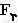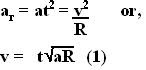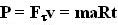## Sunday, February 17, 2008

### Irodov Solution 1.126Let us suppose that thatandare the tangential and radial components of the sum total of all the forces acting on the body. Letandbe the tangential and radial components of the acceleration. Also let v be the tangential velocity of the mass as it moves along the circle. From the problem we know that,This means that,The power generated by all the forces is the derivative of the work done. The motion of the body is always along the tangent of the circle so the only component of force that does any work is the tangential component(the dot product ofand v will be zero) . So the power is given by (see problem 1.125) ,The mean power can simply be determined by integrating over time t and dividing by the time as,Have had also fun trying to solve irodov...while preparing for JEE...so nice to see the solutions online to refer..

great job...

thanks...Anonymous said...

great job...:)

swaroopa said...

superb..... really gr8 job .. thank u..